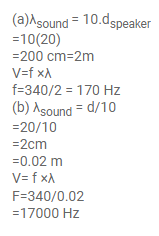# Sound waves from a loudspeaker spread nearly uniformlyQuestion:

Sound waves from a loudspeaker spread nearly uniformly in all directions if the wavelength of the sound is much larger than the diameter of the loudspeaker.

(a)Calculate the frequency for which the wavelength of sound in air is ten times the diameter of the speaker if the diameter is $20 \mathrm{~cm}$.

(b) Sound is essentially transmitted in the forward direction if the wavelength is much shorter than the diameter of the speaker. Calculate the frequency at which the wavelength of the sound is one tenth of the diameter of the speaker described above.

Solution: